Main Content

# Plotting in Polar Coordinates

These examples show how to create line plots, scatter plots, and histograms in polar coordinates. They also show how to annotate and change axes limits on polar plots.

### Create Polar Line Plot

Visualize the radiation pattern from an antenna in polar coordinates. Load the file `antennaData.mat`, which contains the variables `theta` and `rho`. The variable `rho` is a measure of how intensely the antenna radiates for each value of `theta`. Visualize this radiation pattern by plotting the data in polar coordinates using the `polarplot` function.

```load('antennaData.mat') figure polarplot(theta,rho)```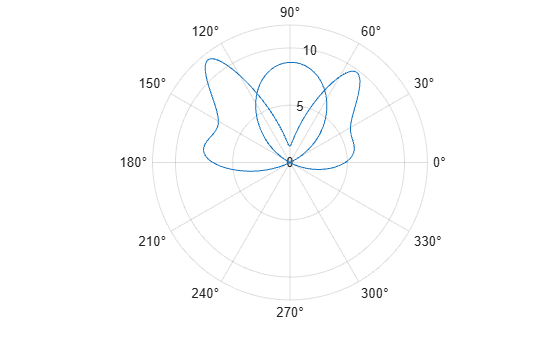### Multiple Polar Line Plots

Use `hold on` to retain the current polar axes and plot additional data using `polarplot`.

```rng('default') noisy = rho + rand(size(rho)); hold on polarplot(theta,noisy) hold off```### Annotating Polar Plots

Use annotation functions such as `legend` and `title` to label polar plots like other visualization types.

```legend('Original','With Noise') title('Antenna Radiation Pattern')```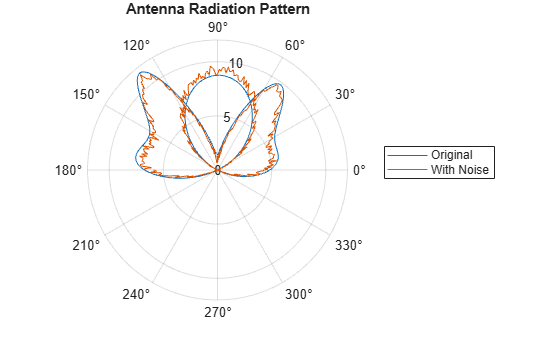### Change Polar Axes Limits

By default, negative values of the radius are plotted as positive values in the polar plot. Use `rlim` to adjust the r-axis limit to include negative values.

```rmin = min(rho); rmax = max(rho); rlim([rmin rmax])```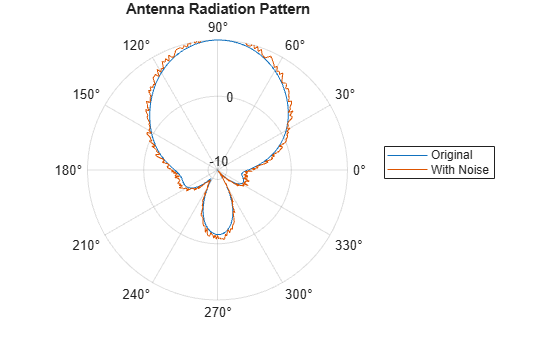Change the theta-axis limits to 0 and 180 with `thetalim`.

`thetalim([0 180])`### Create Polar Scatter Plot

Plot wind velocity data in polar coordinates. Load the file `windData.dat`, which includes the variables `direction`, `speed`, `humidity`, and `C`. Visualize the wind patterns by plotting the data in polar coordinates using the `polarscatter` function.

```load('windData.mat') polarscatter(direction,speed)```Include a third data input to vary marker size and represent a third dimension.

`polarscatter(direction,speed,humidity)`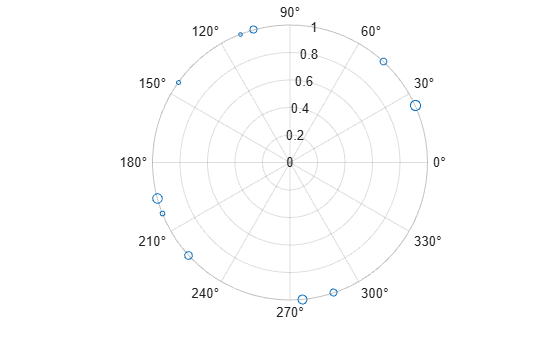Use formatting inputs to adjust marker display properties.

`polarscatter(direction,speed,humidity,C,'filled')`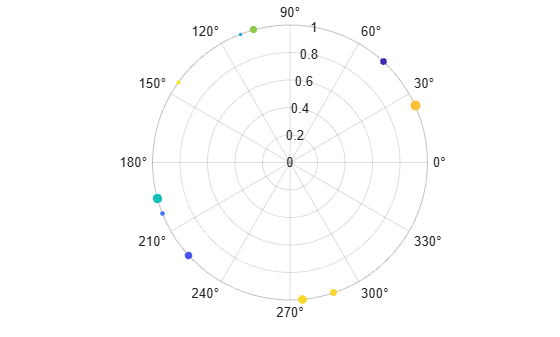### Create Polar Histogram Plot

Visualize the data using the `polarhistogram` function, which produces a visual representation known as a wind rose.

`polarhistogram(direction)`Specify a bin determination algorithm. The `polarhistogram` function has a variety of bin number and bin width determination algorithms to choose from within the `BinMethod` field.

`polarhistogram(direction,'BinMethod','sqrt')`Specify the number of bins and the bin width.

`polarhistogram(direction,24,'BinWidth',.5)`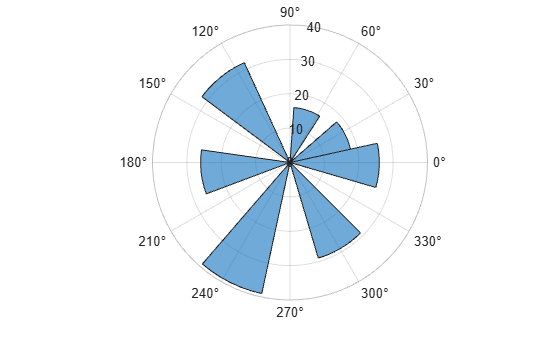Specify a normalization method and adjust the display style to exclude any fill.

`polarhistogram(direction,'Normalization','pdf','DisplayStyle','stairs')`Download ebook